# 149 and Level 2

• 149 is a prime number.
• Prime factorization: 149 is prime.
• The exponent of prime number 149 is 1. Adding 1 to that exponent we get (1 + 1) = 2. Therefore 149 has exactly 2 factors.
• Factors of 149: 1, 149
• Factor pairs: 149 = 1 x 149
• 149 has no square factors that allow its square root to be simplified. √149 ≈ 12.2065556How do we know that 149 is a prime number? If 149 were not a prime number, then it would be divisible by at least one prime number less than or equal to √149 ≈ 12.2. Since 149 cannot be divided evenly by 2, 3, 5, 7, or 11, we know that 149 is a prime number.Excel file of puzzles and previous week’s factor solutions: 12 Factors 2014-06-16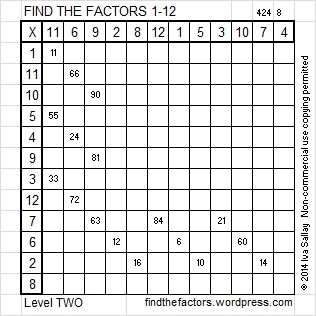# 139 and Level 5

• 139 is a prime number.
• Prime factorization: 139 is prime.
• The exponent of prime number 139 is 1. Adding 1 to that exponent we get (1 + 1) = 2. Therefore 139 has exactly 2 factors.
• Factors of 139: 1, 139
• Factor pairs: 139 = 1 x 139
• 139 has no square factors that allow its square root to be simplified. √139 ≈ 11.789826How do we know that 139 is a prime number? If 139 were not a prime number, then it would be divisible by at least one prime number less than or equal to √139 ≈ 11.8. Since 139 cannot be divided evenly by 2, 3, 5, 7, or 11, we know that 139 is a prime number.

139 is never a clue in the FIND THE FACTORS puzzles.Excel file with puzzles and the previous week’s factor solutions: 12 Factors 2014-06-02# 137 and Level 4

• 137 is a prime number.
• Prime factorization: 137 is prime.
• The exponent of prime number 137 is 1. Adding 1 to that exponent we get (1 + 1) = 2. Therefore 137 has exactly 2 factors.
• Factors of 137: 1, 137
• Factor pairs: 137 = 1 x 137
• 137 has no square factors that allow its square root to be simplified. √137 ≈ 11.7046999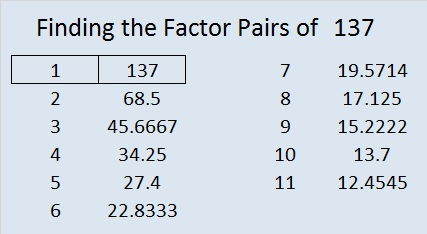How do we know that 137 is a prime number? If 137 were not a prime number, then it would be divisible by at least one prime number less than or equal to √137 ≈ 11.7. Since 137 cannot be divided evenly by 2, 3, 5, 7, or 11, we know that 137 is a prime number.

137 is never a clue in the FIND THE FACTORS puzzles.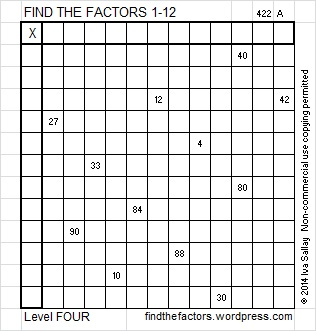Excel file with puzzles and the previous week’s factor solutions: 12 Factors 2014-06-02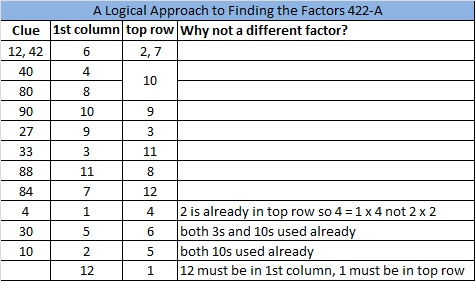# 131 and Level 4

• 131 is a prime number.
• Prime factorization: 131 is prime.
• The exponent of prime number 131 is 1. Adding 1 to that exponent we get (1 + 1) = 2. Therefore 131 has exactly 2 factors.
• Factors of 131: 1, 131
• Factor pairs: 131 = 1 x 131
• 131 has no square factors that allow its square root to be simplified. √131 ≈ 11.4455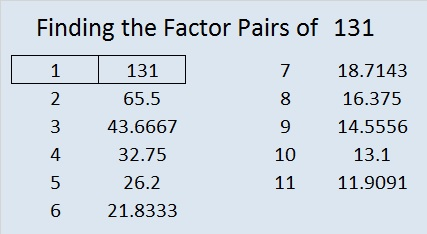How do we know that 131 is a prime number? If 131 were not a prime number, then it would be divisible by at least one prime number less than or equal to √131 ≈ 11.4. Since 131 cannot be divided evenly by 2, 3, 5, 7, or 11, we know that 131 is a prime number.

131 is never a clue in the FIND THE FACTORS puzzles.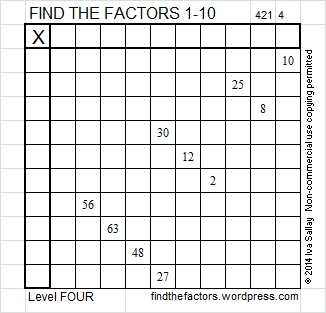Excel file of puzzles and previous week’s solutions: 10 Factors 2014-05-26